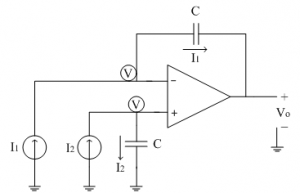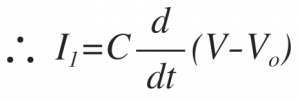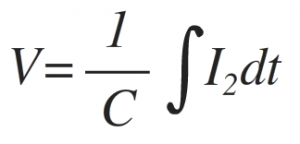Home > analog integrated circuits > op amp integrator > difference integral

# Difference integral

The following figure shows the configuration of op-amp as a difference (differential) integrator. Here inputs are two currents I1 and I2.Let us assume that the inverting terminal of op-amp is at potential 'V'. Due to virtual ground concept the non-inverting terminal also appears to be at same potential 'V'
The current I1 flows through the feedback capacitance C because input current to op-amp is zero.Taking integration on both the sides, we get,At non-inverting terminal we can have,Substituting this V in above output equation, we get,Thus output is integral of difference of two input currents I1 and I2. Thus acts as difference integrator.